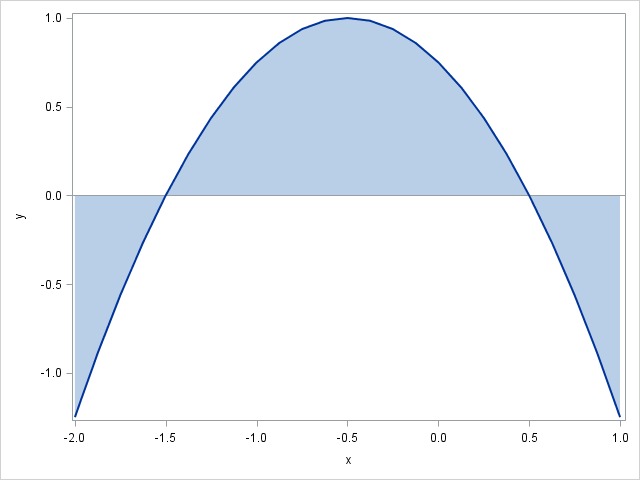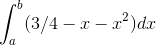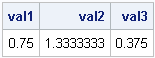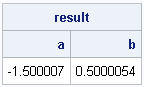Last week I showed how to find parameters that maximize the integral of a certain probability density function (PDF). Because the function was a PDF, I could evaluate the integral by calling the CDF function in SAS. (Recall that the cumulative distribution function (CDF) is the integral of a PDF.)

You can also use SAS to optimize an integral function when the integrand is not a PDF. This follow-up article shows how to optimize the integral of an arbitrary function by calling the QUAD subroutine in SAS/IML software to evaluate the integral.

### Defining an integralThis example finds parameters (a,b) such that the integral of the f(x) = 3/4 – xx2 is the maximum on the interval [a, b]. That is, the problem is to maximizesubject to the constraint that a < b.

The graph of the integrand is shown at the left. The parameters determine the domain of integration. The graph shows the integral (area under the curve) for the values (a,b)=(–2,1). From the graph, you can see that the optimal parameters are (a,b) =(–1.5, 0.5), which integrates the quadratic function over the interval on which it is positive. (Because the function is negative outside of (–1.5, 0.5), we don't want to accumulate "negative area.")

To solve this problem in the SAS/IML language, you need to define the integrand and call the QUAD function to evaluate the integral, as follows:

```proc iml; /* Define the integrand, which will be called by QUAD subroutine. For this problem, the integrand does not depend on the parameters, but for other problems, it might. */ start Func(x); /* use GLOBAL stmt if Func depends on parameters */ return( 3/4 - x - x##2 ); finish;   /* Define a function that computes the integral. This will be called by an NLP routine, so the function must depend on the parameters. */ start Integral(parms); /* use GLOBAL stmt if Func depends on parameters */ /* the parameters are: a = parms; b = parms; */ call quad(val, "Func", parms); /* evaluate integral on [a, b] */ return( val ); finish;```

The module named Integral defines the objective function, which will be maximized by calling one of the NLP functions in SAS/IML software. However, you should NEVER start optimizing until you have verified that the objective function is defined correctly! The following statements test the objective function by evaluating the integral on three different domains. Because this quadratic function has a simple antiderivative, you can verify analytically that the Integral function is performing the integration correctly:

```/* Check and debug objective function BEFORE using it in NLP! */ val1 = Integral({-2 1}); val2 = Integral({-1.5 0.5}); val3 = Integral({-0.5 1}); print val1 val2 val3;```### Defining the gradient vector of derivatives

One of my favorite nonlinear optimization methods is the Newton-Raphson algorithm, which is implemented in SAS/IML as the NLPNRA subroutine. This algorithm uses derivatives of the objective function to help find the maximal value of the function. The algorithm will compute finite-difference derivatives if you do not supply an analytic derivative function, but in this example it is trivial to define the gradient vector, which is the vector of derivatives of the objective function with respect to the parameters. Because the parameters are the upper and lower limits of integration, the fundamental theorem of calculus tells us that the derivatives for this problem are found by evaluating the integrand at the parameter values:

```/* Optional: Define derivative w.r.t. parameters. By the FTC, the derivative is found by evaluating the integrand at the parameters. */ start Deriv(parms); dfda = -Func( parms ); /* deriv at lower limit of integration */ dfdb = Func( parms ); /* deriv at upper limit of integration */ return( dfda || dfdb ); finish;```

### Maximizing the objective function

This maximization problem is subject to the constraint that a < b. You can specify boundary and linear constraints by using the BLC= option to the NLP subroutines. You can use the following matrix to specify the constraint to an NLP function:

```/* Set up linear constraint a < b, which is also a - b < 0 */ /* a b OP val */ con = {. . . ., /* no constraint on lower bound of a or b */ . . . ., /* no constraint on upper bound of a or b */ 1 -1 -1 0}; /* 1*a - 1*b <= 0 */```

Finally, you can specify an initial guess and call the NLPNRA subroutine to find the parameter values that maximize the integral:

```p = {-1 1}; /* initial guess for solution */ opt = {1, /* find maximum of function */ 2}; /* print a little bit of output */ call nlpnra(rc, result, "Integral", p, opt) blc=con jac="Deriv"; print result[c={"a" "b"}];```The result agrees with our intuition. The Newton-Raphson algorithm determined that the interval(a,b) = (–1.5, 0.5) maximizes the integral of the quadratic function. The maximal value of the integral is 4/3.

• Optimize a function that depends on one or more parameters. The "twist" is that the objective function requires evaluating an integral.
• Use the QUAD subroutine to numerically compute the integral an arbitrary function.
• Specify linear constraints in an optimization problem.

ShareDistinguished Researcher in Computational Statistics

Rick Wicklin, PhD, is a distinguished researcher in computational statistics at SAS and is a principal developer of SAS/IML software. His areas of expertise include computational statistics, simulation, statistical graphics, and modern methods in statistical data analysis. Rick is author of the books Statistical Programming with SAS/IML Software and Simulating Data with SAS.

1.